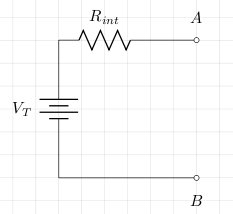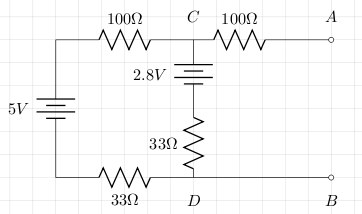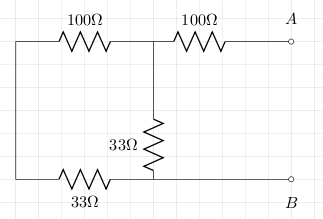# Thevenin's Theorem

Thevenin's theorem states that any two terminal voltage source can be represented by a single voltage source with internal resistance Rint as in the circuit below:The values for VT and Rint can either be calculated or measured.

### Calculation

When calculating, first use Kirchhoff's or superposition to compute the open circuit voltage VT. Next calculate the value of Rint by replacing all voltage sources by their internal resistances.

### Measuring

To measure, first find the short circuit current from A to B and call this ISC. Next measure the open circuit voltage (VT). The value of Rint is VT/ISC.

## Example

We'll calculate and measure the values for VT and Rint for the circuit below.### Calculation

We can use Kirchhoff's voltage law to give us the current:

```5 - 2.8 = I*(100+33+33)
2.2 = I*166.
I = 2.2/166 = 0.013
```

Now VT is VCD, so:
VT = 2.8 + 0.013*33 = 3.23

For Rint we short all voltage sources (assuming no internal resistance) and this gives the following circuit:The calculation of Rint is quite easy:
Rint = 100 + 1/(1/33 + 1/133) = 100 + 26.4 = 126.4

From this we can calculate the short circuit current:
ISC = 3.23 / 126.4 = 0.0255.

### Measurement

The circuit was built and using a multimeter the value of VT(VCD) was 3.3V. The short circuit current ISC was measured to be 25.6mA. From this we can calculate Rint:
Rint = 3.3/0.0256 = 128.9

### Comparison

The table below shows how close the actual measurements were to the theoretical calculations.

VT ISC Rint 3.3 0.0256 128.9 3.23 0.0255 126.4

## References

Fischer-Cripps. A.C., The Electronics Companion. Institute of Physics, 2005.